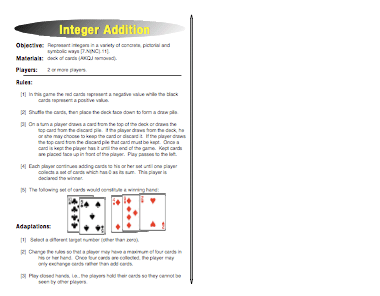Integer Addition: Integer GameThe Integer Addition Game does something very interesting. It uses the red cards for a negative value and the black cards for a positive value. This is a great strategy since the phrase “in the red” means a company is losing money and the phrase “in the black” means that it’s making money. If you use those phrases, this company would be “breaking even.” By playing this game, students develop an understanding of what it means for two numbers to be additive inverses of each other. The numbers essentially cancel each other out to yield a sum of zero. There’s some strategy and risk in the game as well since students have to figure out whether they want to draw from the deck or accept the card they are dealt from the discard pile. The luck of the draw might mean that they pick a number that’s perfect to yield the final sum of zero or one that takes them far away from the correct combination.

Many kids have trouble conceptualizing integers but by using this model it will help them to understand the significance of positive and negative numbers. Once students have the additive inverses down pat, you can also ask them to create sums and differences to yield certain numbers. For example, you might ask them to figure out a combination that would yield negative 7 when added. Another adaptation is to assign values to the Jack, Queen, and King cards so that they can be added to the game to provide even more challenge.

Negative and positive symbols on a piece of paper will have a lot more meaning to students after they have played this game. You might want to ask them to write their hand of cards as an expression to see if they can translate the model they are seeing in the cards to a viable expression that represents the correct numbers.

Common Core Mathematical Standard
7.NS Apply and extend previous understandings of operations with fractions to add, subtract, multiply, and divide rational numbers.

Common Core Standards for Mathematical Practice
4. Model with mathematics.

Focus On Integers  – ULTIMATE Resource!Check out this bundle here

This site uses Akismet to reduce spam. Learn how your comment data is processed.